10 Fixed-Axis Rotation

10.3 Relating Angular and Translational Quantities

Learning Objectives

By the end of this section, you will be able to:

• Given the linear kinematic equation, write the corresponding rotational kinematic equation
• Calculate the linear distances, velocities, and accelerations of points on a rotating system given the angular velocities and accelerations

In this section, we relate each of the rotational variables to the translational variables defined in Motion Along a Straight Line and Motion in Two and Three Dimensions. This will complete our ability to describe rigid-body rotations.

Angular vs. Linear Variables

In Rotational Variables, we introduced angular variables. If we compare the rotational definitions with the definitions of linear kinematic variables from Motion Along a Straight Line and Motion in Two and Three Dimensions, we find that there is a mapping of the linear variables to the rotational ones. Linear position, velocity, and acceleration have their rotational counterparts, as we can see when we write them side by side:

Linear Rotational
Position x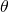Velocity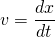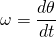Acceleration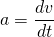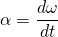Let’s compare the linear and rotational variables individually. The linear variable of position has physical units of meters, whereas the angular position variable has dimensionless units of radians, as can be seen from the definition of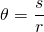, which is the ratio of two lengths. The linear velocity has units of m/s, and its counterpart, the angular velocity, has units of rad/s. In Rotational Variables, we saw in the case of circular motion that the linear tangential speed of a particle at a radius r from the axis of rotation is related to the angular velocity by the relation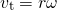. This could also apply to points on a rigid body rotating about a fixed axis. Here, we consider only circular motion. In circular motion, both uniform and nonuniform, there exists a centripetal acceleration (Motion in Two and Three Dimensions). The centripetal acceleration vector points inward from the particle executing circular motion toward the axis of rotation. The derivation of the magnitude of the centripetal acceleration is given in Motion in Two and Three Dimensions. From that derivation, the magnitude of the centripetal acceleration was found to be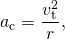where r is the radius of the circle.

Thus, in uniform circular motion when the angular velocity is constant and the angular acceleration is zero, we have a linear acceleration—that is, centripetal acceleration—since the tangential speed in (Figure) is a constant. If nonuniform circular motion is present, the rotating system has an angular acceleration, and we have both a linear centripetal acceleration that is changing (because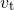is changing) as well as a linear tangential acceleration. These relationships are shown in (Figure), where we show the centripetal and tangential accelerations for uniform and nonuniform circular motion.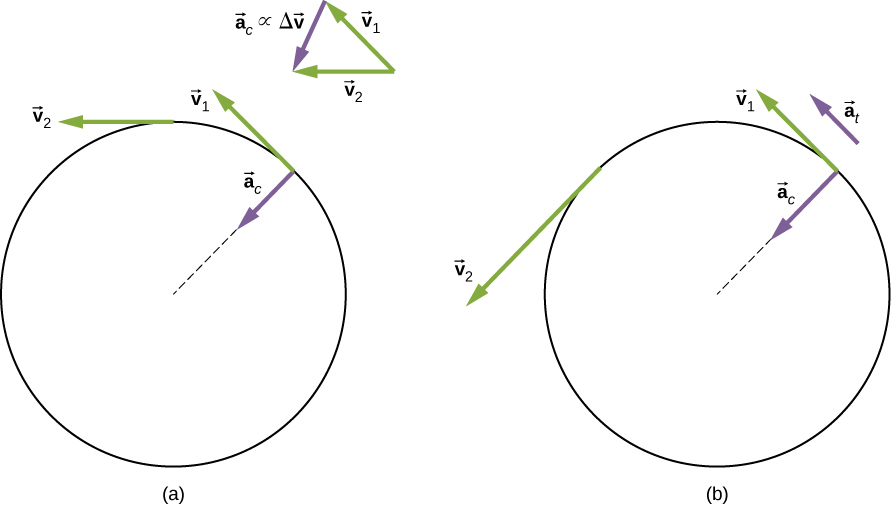Figure 10.14 (a) Uniform circular motion: The centripetal acceleration     has its vector inward toward the axis of rotation. There is no tangential acceleration. (b) Nonuniform circular motion: An angular acceleration produces an inward centripetal acceleration that is changing in magnitude, plus a tangential acceleration     .

The centripetal acceleration is due to the change in the direction of tangential velocity, whereas the tangential acceleration is due to any change in the magnitude of the tangential velocity. The tangential and centripetal acceleration vectors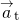and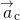are always perpendicular to each other, as seen in (Figure). To complete this description, we can assign a total linear acceleration vector to a point on a rotating rigid body or a particle executing circular motion at a radius r from a fixed axis. The total linear acceleration vector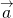is the vector sum of the centripetal and tangential accelerations,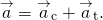The total linear acceleration vector in the case of nonuniform circular motion points at an angle between the centripetal and tangential acceleration vectors, as shown in (Figure). Since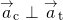, the magnitude of the total linear acceleration isNote that if the angular acceleration is zero, the total linear acceleration is equal to the centripetal acceleration.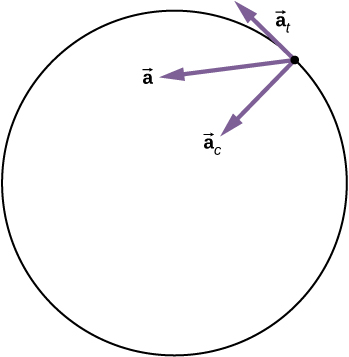Figure 10.15 A particle is executing circular motion and has an angular acceleration. The total linear acceleration of the particle is the vector sum of the centripetal acceleration and tangential acceleration vectors. The total linear acceleration vector is at an angle in between the centripetal and tangential accelerations.

Relationships between Rotational and Translational Motion

We can look at two relationships between rotational and translational motion.

1. Generally speaking, the linear kinematic equations have their rotational counterparts. (Figure) lists the four linear kinematic equations and the corresponding rotational counterpart. The two sets of equations look similar to each other, but describe two different physical situations, that is, rotation and translation.
Rotational and Translational Kinematic Equations
Rotational Translational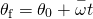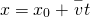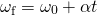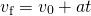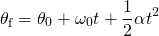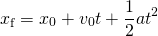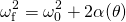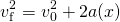2. The second correspondence has to do with relating linear and rotational variables in the special case of circular motion. This is shown in (Figure), where in the third column, we have listed the connecting equation that relates the linear variable to the rotational variable. The rotational variables of angular velocity and acceleration have subscripts that indicate their definition in circular motion.
Rotational and Translational Quantities: Circular Motion
Rotational Translational Relationship (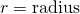)s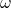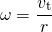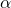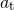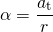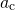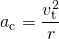Example

Linear Acceleration of a Centrifuge

A centrifuge has a radius of 20 cm and accelerates from a maximum rotation rate of 10,000 rpm to rest in 30 seconds under a constant angular acceleration. It is rotating counterclockwise. What is the magnitude of the total acceleration of a point at the tip of the centrifuge at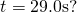What is the direction of the total acceleration vector?

Strategy

With the information given, we can calculate the angular acceleration, which then will allow us to find the tangential acceleration. We can find the centripetal acceleration at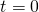by calculating the tangential speed at this time. With the magnitudes of the accelerations, we can calculate the total linear acceleration. From the description of the rotation in the problem, we can sketch the direction of the total acceleration vector.

Solution

The angular acceleration is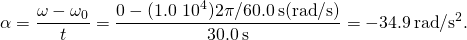Therefore, the tangential acceleration is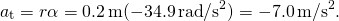The angular velocity at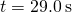is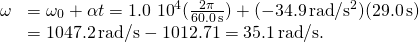Thus, the tangential speed atis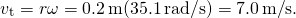We can now calculate the centripetal acceleration at: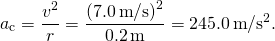Since the two acceleration vectors are perpendicular to each other, the magnitude of the total linear acceleration is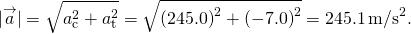Since the centrifuge has a negative angular acceleration, it is slowing down. The total acceleration vector is as shown in (Figure). The angle with respect to the centripetal acceleration vector is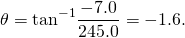The negative sign means that the total acceleration vector is angled toward the clockwise direction.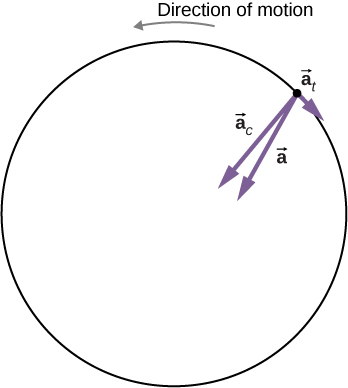Figure 10.16 The centripetal, tangential, and total acceleration vectors. The centrifuge is slowing down, so the tangential acceleration is clockwise, opposite the direction of rotation (counterclockwise).

Significance

From (Figure), we see that the tangential acceleration vector is opposite the direction of rotation. The magnitude of the tangential acceleration is much smaller than the centripetal acceleration, so the total linear acceleration vector will make a very small angle with respect to the centripetal acceleration vector.

A boy jumps on a merry-go-round with a radius of 5 m that is at rest. It starts accelerating at a constant rate up to an angular velocity of 5 rad/s in 20 seconds. What is the distance travelled by the boy?

The angular acceleration is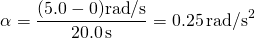. Therefore, the total angle that the boy passes through is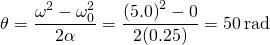.

Thus, we calculate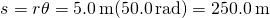.

Check out this PhET simulation to change the parameters of a rotating disk (the initial angle, angular velocity, and angular acceleration), and place bugs at different radial distances from the axis. The simulation then lets you explore how circular motion relates to the bugs’ xy-position, velocity, and acceleration using vectors or graphs.

Summary

• The linear kinematic equations have their rotational counterparts such that there is a mapping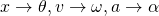.

• A system undergoing uniform circular motion has a constant angular velocity, but points at a distance r from the rotation axis have a linear centripetal acceleration.
• A system undergoing nonuniform circular motion has an angular acceleration and therefore has both a linear centripetal and linear tangential acceleration at a point a distance r from the axis of rotation.
• The total linear acceleration is the vector sum of the centripetal acceleration vector and the tangential acceleration vector. Since the centripetal and tangential acceleration vectors are perpendicular to each other for circular motion, the magnitude of the total linear acceleration is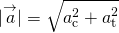.

Conceptual Questions

Explain why centripetal acceleration changes the direction of velocity in circular motion but not its magnitude.

The centripetal acceleration vector is perpendicular to the velocity vector.

In circular motion, a tangential acceleration can change the magnitude of the velocity but not its direction. Explain your answer.

Suppose a piece of food is on the edge of a rotating microwave oven plate. Does it experience nonzero tangential acceleration, centripetal acceleration, or both when: (a) the plate starts to spin faster? (b) The plate rotates at constant angular velocity? (c) The plate slows to a halt?

a. both; b. nonzero centripetal acceleration; c. both

Problems

At its peak, a tornado is 60.0 m in diameter and carries 500 km/h winds. What is its angular velocity in revolutions per second?

A man stands on a merry-go-round that is rotating at 2.5 rad/s. If the coefficient of static friction between the man’s shoes and the merry-go-round is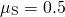, how far from the axis of rotation can he stand without sliding?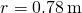An ultracentrifuge accelerates from rest to 100,000 rpm in 2.00 min. (a) What is the average angular acceleration in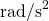? (b) What is the tangential acceleration of a point 9.50 cm from the axis of rotation? (c) What is the centripetal acceleration in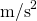and multiples of g of this point at full rpm? (d) What is the total distance travelled by a point 9.5 cm from the axis of rotation of the ultracentrifuge?

A wind turbine is rotating counterclockwise at 0.5 rev/s and slows to a stop in 10 s. Its blades are 20 m in length. (a) What is the angular acceleration of the turbine? (b) What is the centripetal acceleration of the tip of the blades at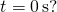(c) What is the magnitude and direction of the total linear acceleration of the tip of the blades ata.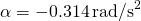,
b.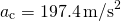; c.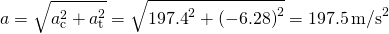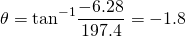in the clockwise direction from the centripetal acceleration vector

What is (a) the angular speed and (b) the linear speed of a point on Earth’s surface at latitude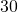N. Take the radius of the Earth to be 6309 km. (c) At what latitude would your linear speed be 10 m/s?

A child with mass 30 kg sits on the edge of a merry-go-round at a distance of 3.0 m from its axis of rotation. The merry-go-round accelerates from rest up to 0.4 rev/s in 10 s. If the coefficient of static friction between the child and the surface of the merry-go-round is 0.6, does the child fall off before 5 s?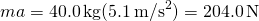The maximum friction force is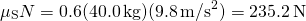so the child does not fall off yet.

A bicycle wheel with radius 0.3m rotates from rest to 3 rev/s in 5 s. What is the magnitude and direction of the total acceleration vector at the edge of the wheel at 1.0 s?

The angular velocity of a flywheel with radius 1.0 m varies according to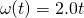. Plot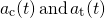from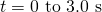for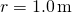. Analyze these results to explain when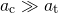and when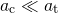for a point on the flywheel at a radius of 1.0 m.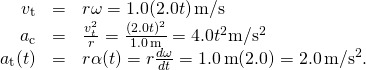Plotting both accelerations gives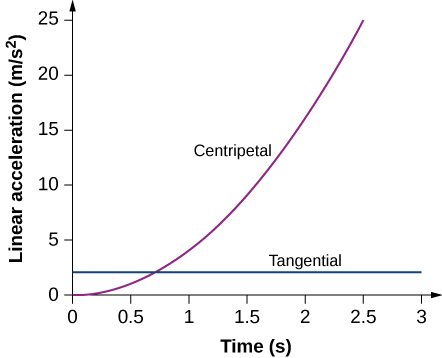The tangential acceleration is constant, while the centripetal acceleration is time dependent, and increases with time to values much greater than the tangential acceleration after t = 1s. For times less than 0.7 s and approaching zero the centripetal acceleration is much less than the tangential acceleration.